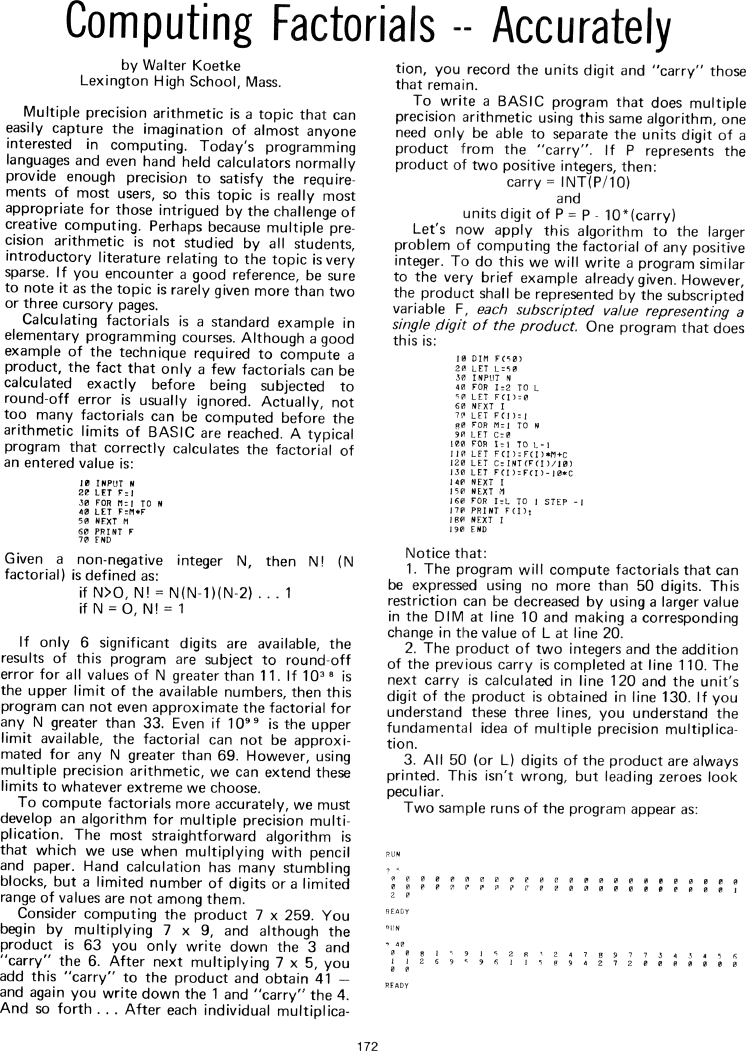The Best of Creative Computing Volume 1 (published 1976)

 `Page 172 << PREVIOUS >> NEXT Jump to page: Go to contents Go to thumbnails`

Computing Factorials Accurately```Computing Factorials -- Accurately

by Walter Koetke
Lexington High School, Mass.

Multiple precision arithmetic is a topic that can easily capture the imagination
of almost anyone interested in computing.Today's programming languages and even
hand held calculators normally provide enough precision to satisfy the
requirements of most users, so this topic is really most appropriate for those
intrigued by the challenge of creative computing. Perhaps because multiple
precision arithmetic is not studied by all students,
introductory literature relating to the topic is very sparse.If you encounter a
good reference, be sure to note it as the topic is rarely given more than two or
three cursory pages.

Calculating factorials is a standard example in elementary programming courses.
Although a good example of the technique required to compute a product, the fact
that only a few factorials can be calculated exactly before being subjected to
round-off error is usually ignored. Actually, not too many factorials can be
computed before the arithmetic limits of BASIC are reached. A typical program
that correctly calculates the factorial of an entered value is:

10 INPUT N
20 LET F = 1
30 FOR M = 1 TO N
40 LET F = M*F
50 NEXT M
60 PRINT F
70 END
Given a non-negative integer N, then N! (N factorial) is defined as:

if N>O, N! = N(N-1)(N-2) . . .1
if N = O, N! = 1

If only 6 significant digits are available, the results of this program are
subject to round-off error for all values of N greater than 11. If 10" is the
upper limit of the available numbers, then this program can not even approximate
the factorial for any N greater than 33. Even if 10" is the upper limit
available, the factorial can not be approximated for any N greater than 69.
However, using multiple precision arithmetic, we can extend these limits to
whatever extreme we choose.

To compute factorials more accurately, we must develop an algorithm for multiple
precision multiplication. The most straightforward algorithm is that which we
use when multiplying with pencil and paper. Hand calculation has many stumbling
blocks, but a limited number of digits or a limited range of values are not
among them.

Consider computing the product 7 x 259. You begin by multiplying 7 x 9, and
although the product is 63 you only write down the 3 and "carry" the 6. After
next multiplying 7 x 5, you add this "carry" to the product and obtain 41 and
again you write down the 1 and "carry" the 4.And so forth _ _ _ After each
individual multiplication, you record the units digit and "carry" those that
remain.

To write a BASIC program that does multiple precision arithmetic using this same
algorithm, one need only be able to separate the units digit of a product from
the "carry". lf P represents the product of two positive integers, then:

carry = INT(P/10)
and
units digit of P = P -10*(carry)

Let's now apply this algorithm to the larger problem of computing the factorial
of any positive integer. To do this we will write a program similar to the very
brief example already given. However,the product shall be represented by the
subscripted variable F, each subscripted value representing a single digit of
the product. One program that does this is:

10 DIM F(50)
20 LET L=50
30 INPUT N
40 FOR I=2 TO L
50 LET F(I)=0
60 NFXT I
70 LET F(1)= 1
80 FOR M=l T0 N
90 LET C=0
100 FOR I=1 T0 L-l
ll0 LET F(I)=F(I)*M+C
120 LET C=INT(F(I)/10)
130 LET F(I)=F(I)-10*C
140 NEXT I
150 NEXT M
160 FOR I=L TO 1 STEP -1
170 PRINT F(I):

180 NEXT I
190 END

Notice that:

1. The program will compute factorials that can be expressed using no more than
50 digits. This restriction can be decreased by using a larger value in the DIM
at line 10 and making a corresponding
change in the value of L at line 20.

2. The product of two integers and the addition of the previous carry is
completed at line 110. The next carry is calculated in line 120 and the unit's
digit of the product is obtained in line 130. If you understand these three
lines, you understand the fundamental idea of multiple precision multiplication.

3. All 50 (or L) digits of the product are always printed. This isn't wrong, but

Two sample runs of the program appear as:

RUN

0 0 0 0 0 0 0 0 0 0 0 0 0 0 0 0 0 0 0 0 0 0 0 0
0 0 0 0 0 0 0 0 0 0 0 0 0 0 0 0 0 0 0 0 0 0 0 1
2 0

RUN

0 0 8 1 5 9 1 5 2 8 3 2 4 7 8 9 7 7 3 4 3 4 5 6
1 1 2 6 9 5 9 6 1 1 5 8 9 4 2 7 2 0 0 0 0 0 0 0
0 0

<!--